### Archive

Posts Tagged ‘Video Games’

## Counting and Solving Final Fantasy XIII-2’s Clock Puzzles

February 6th, 2012

Final Fantasy XIII-2 is a role-playing game, released last week in North America, that contains an abundance of mini-games. One of the more interesting mini-games is the “clock puzzle”, which presents the user with N integers arranged in a circle, with each integer being from 1 to$\lfloor N/2 \rfloor$.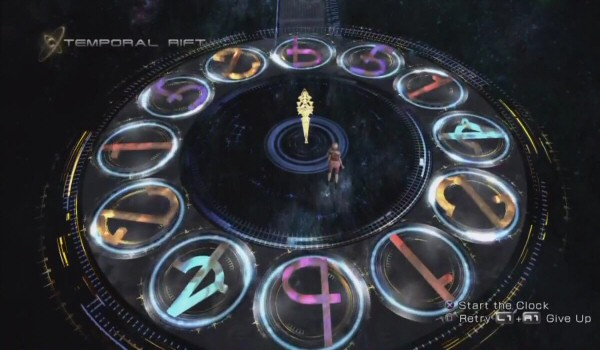A challenging late-game clock puzzle with N = 12

The way the game works is as follows:

1. The user may start by picking any of the N positions on the circle. Call the number in this position M.
2. You now have the option of picking either the number M positions clockwise from your last choice, or M positions counter-clockwise from your last choice. Update the value of M to be the number in the new position that you chose.
3. Repeat step 2 until you have performed it N-1 times.

You win the game if you choose each of the N positions exactly once, and you lose the game otherwise (if you are forced to choose the same position twice, or equivalently if there is a position that you have not chosen after performing step 2 a total of N-1 times). During the game, N ranges from 5 to 13, though N could theoretically be as large as we like.

### Example

To demonstrate the rules in action, consider the following simple example with N = 6 (I have labelled the six positions 05 in blue for easy reference):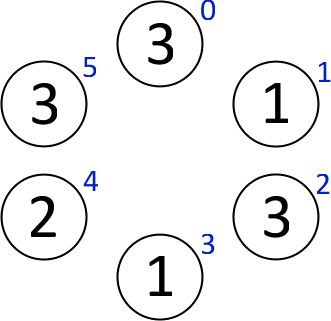If we start by choosing the 1 in position 1, then we have the option of choosing the 3 in either position 0 or 2. Let’s choose the 3 in position 0. Three moves either clockwise or counter-clockwise from here both give the 1 in position 3, so that is our only possible next choice. We continue on in this way, going through the N = 6 positions in the order 103425, as in the following image: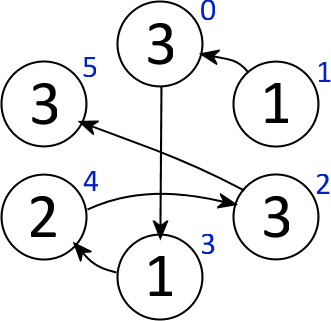We have now selected each position exactly once, so we are done – we solved the puzzle! In fact, this is the unique solution for the given puzzle.

### Counting Clock Puzzles

Let’s work on determining how many different clock puzzles there are of a given size. As mentioned earlier, a clock puzzle with N positions has an integer in the interval$[1, \lfloor N/2 \rfloor]$ in each of the  positions. There are thus$\lfloor N/2 \rfloor^N$ distinct clock puzzles with N positions, which grows very quickly with N – its values for N = 1, 2, 3, … are given by the sequence 0, 1, 1, 16, 32, 729, 2187, 65536, 262144, … (A206344 in the OEIS).

However, this rather crude count of the number of clock puzzles ignores the fact that some clock puzzles have no solution. To illustrate this fact, we present the following simple proposition:

Proposition. There are unsolvable clock puzzles with N positions if and only if N = 4 or N ≥ 6.

To prove this proposition, first note that the clock puzzles for N = 2 or N = 3 are trivially solvable, since each number in the puzzle is forced to be$\lfloor N/2 \rfloor = 1$. The 32 clock puzzles in the N = 5 case can all easily be shown to be solvable via computer brute force (does anyone have a simple or elegant argument for this case?).

In the N = 4 case, exactly 3 of the 16 clock puzzles are unsolvable: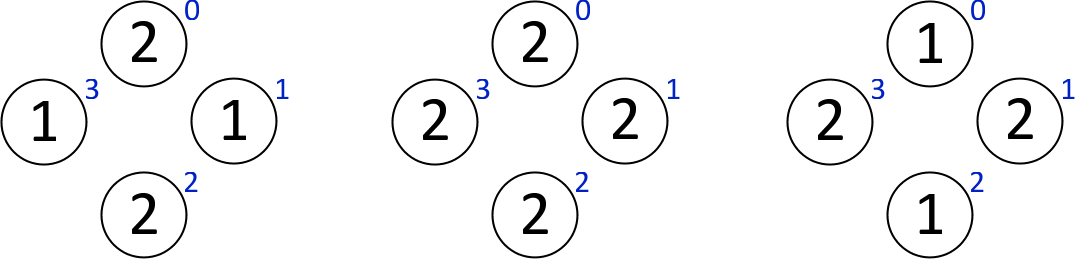To complete the proof, it suffices to demonstrate an unsolvable clock puzzle for each N ≥ 6. To this end, we begin by considering the following clock puzzle in the N = 6 case: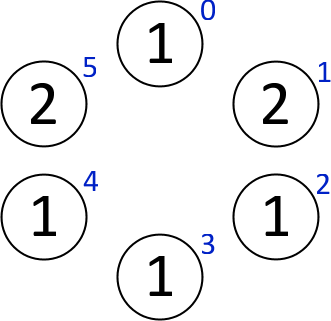The above puzzle is unsolvable because the only way to reach position 0 is to select it first, but from there only one of positions 2 or 4 can be reached – not both. This example generalizes in a straightforward manner to any N ≥ 6 simply by adding more 1’s to the bottom: it will still be necessary to choose position 0 first, and then it is impossible to reach both position 2 and position N-2 from there.

There doesn’t seem to be an elegant way to count the number of solvable clock puzzles with N positions (which is most likely related to the apparent difficulty of solving these puzzles, which will be discussed in the next section), so let’s count the number of solvable clock puzzles via brute force. Simply constructing each of the$\lfloor N/2 \rfloor^N$ clock puzzles and determining which of them are solvable (via the MATLAB script linked at the end of this post) shows that the number of solvable clock puzzles for N = 1, 2, 3, … is given by the sequence 0, 1, 1, 13, 32, 507, 1998, 33136, 193995, … (A206345 in the OEIS).

This count of puzzles is perhaps still unsatisfying, though, since it counts puzzles that are simply mirror images or rotations of each other multiple times. Again, there doesn’t seem to be an elegant counting argument for enumerating the solvable clock puzzles up to rotation and reflection, so we compute this sequence by brute force: 0, 1, 1, 4, 8, 72, 236, 3665, 19037, … (A206346 in the OEIS).

### Solving Clock Puzzles

Clock puzzles are one of the most challenging parts of Final Fantasy XIII-2, and with good reason: they are a well-studied graph theory problem in disguise. We can consider each clock puzzle with N positions as a directed graph with N vertices. If position N contains the number M, then there is a directed edge going from vertex N to the vertices M positions clockwise and counter-clockwise from it. In other words, we consider a clock puzzle as a directed graph on N vertices, where the directed edges describe the valid moves around the circle.The directed graph corresponding to the earlier (solvable) N = 6 example

The problem of solving a clock puzzle is then exactly the problem of finding a directed Hamiltonian path on the associated graph. Because finding a directed Hamiltonian path in general is NP-hard, this seems to suggest that solving clock puzzles might be as well. There of course is the problem that the directed graphs relevant to this problem have very special structure – in particular, every vertex has outdegree ≤ 2, and the graph has a symmetry property that results from clockwise/counter-clockwise movement allowed in the clock puzzles.

The main result of  shows that the fact that the outdegree of each vertex is no larger than 2 is no real help: finding directed Hamiltonian paths is still NP-hard given such a promise. However, the symmetry condition seems more difficult to characterize in graph theoretic terms, and could potentially be exploited to produce a fast algorithm for solving these puzzles.

Regardless of the problem’s computational complexity, the puzzles found in the game are quite small (N ≤ 13), so they can be easily solved by brute force. Attached is a MATLAB script (solve_clock.m) that can be used to solve clock puzzles. The first input argument is a vector containing the numeric values in each of the positions, starting from the top and reading clockwise. By default, only one solution is computed. To compute all solutions, set the second (optional) input argument to 1.

The output of the script is either a vector of positions (labelled 0 through N-1, with 0 referring to the top position, 1 referring to one position clockwise from there, and so on) describing an order in which you can visit the positions to solve the puzzle, or 0 if there is no solution.

For example, the script can be used to find our solution to the N = 6 example provided earlier:

```>> solve_clock([3,1,3,1,2,3])

ans =
1 0 3 4 2 5```

Similarly, the script can be used to find all four solutions [Update, October 1, 2013: Whoops, there are six solutions! See the comments.] to the puzzle in the screenshot at the very top of this post:

```>> solve_clock([6,5,1,4,2,1,6,4,2,1,5,2], 1)

ans =
3 7 11 9 10 5 4 2 1 8 6 0
7 3 11 9 10 5 4 2 1 8 6 0
9 10 5 4 2 3 7 11 1 8 6 0
9 8 10 5 4 2 3 7 11 1 6 0```